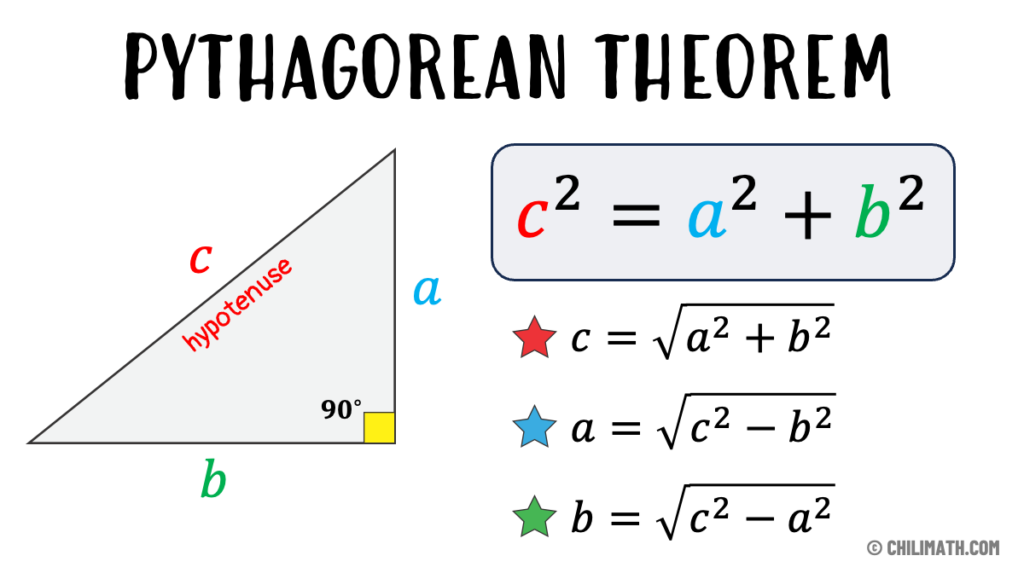I was moving some furniture this weekend and I encountered an age-old geometric dilemma – how to easily estimate the diagonal length of square floor tiles.

For centuries, we’ve all been made to memorize a² + b² = c² to compute hypotenuses of right triangles. But when I needed to quickly estimate diagonal lengths while moving furniture, using this formula while holding a couch was a real pain.

The Pythagorean Theorem is one of the oldest and most famous mathematical formulas in history. It states that in any right triangle, the square of the hypotenuse (the longest side) is equal to the sum of the squares of the other two sides. This theorem was discovered by many ancient civilizations, such as the Babylonians, Egyptians, Indians, and Chinese, but it is named after the Greek philosopher and mathematician Pythagoras, who proved it around 500 BC. The Pythagorean Theorem has many applications in geometry, algebra, trigonometry, physics, engineering, and other fields of science. It can be used to find the length of any side of a right triangle, to calculate the distance between two points, to check if a triangle is right-angled or not, and to construct various shapes and patterns.

However, the Pythagorean Theorem also has some drawbacks for practical use. First of all, it requires knowing or measuring two sides of a right triangle accurately, which may not be always possible or convenient. Second of all, it involves squaring and adding numbers, which may not be easy to do mentally or visually. Third of all, it involves taking the square root of a number, which may not be an integer or a simple fraction. Taking the square root of a number can be cumbersome and unintuitive, especially if the number is large or has many digits.While staring at square floor tiles and working out the square roots and their sums, I realized that, if you bisect a square along its diagonal, you get two right triangles whose hypotenuse is equal to the diagonal of the square. Now, if you look at these triangles closely, you will notice that their hypotenuse is very close to 1.4 times the length of one side of the square. In fact, the exact ratio is √2 ≈ 1.414 , which is only 1% more than 1.4 . This means that if you know or estimate the length of one side of a square floor tile, you can easily multiply it by 1.4 to get an approximate diagonal length.

For example, suppose you have a square floor tile that measures 10 inches on each side. To find its diagonal length using the Pythagorean Theorem, you would have to do this calculation: √(10² + 10² ) = √200 ≈ 14.142 inches. To find its diagonal length using my rule of thumb, you would just have to do this calculation: 10 x 1.4 = 14 inches. As you can see, my rule of thumb gives a very close estimate with much less effort.

Here is another example: suppose you have a square floor tile that measures 5 centimeters on each side. To find its diagonal length using the Pythagorean Theorem, you would have to do this calculation: √(5² + 5² ) = √50 ≈ 7.071 centimeters. To find its diagonal length using my rule of thumb, you would just have to do this calculation: 5 x 1.4 = 7 centimeters. Again, my rule of thumb gives a very close estimate with much less effort.It does not require knowing or measuring two sides of a right triangle, only one. It does not involve squaring and adding numbers, only multiplying by 1.4 . It does not involve taking the square root of a number, only rounding to the nearest integer or fraction. Therefore, if you need a quick and easy estimate or a visual approximation for any square floor tile, you should use my rule of thumb instead of the Pythagorean Theorem.

I was sure someone else must have thought of this in the past 2,500 years, but no – I could find no reference whatsoever to this formula. So, in all modesty, I will have to claim it!

Interior designers, carpenters, geometricians and kids taking multiple choice tests can ditch the calculator and just remember the “Davis 140% Diagonal Rule” instead. While not mathematically precise, my shortcut blows Pythagoras out of the water for practical usability. Sometimes close enough is all you need.

I’m thrilled to present this discovery that upgrades an ancient formula for modern simplicity. The diagonal of a square’s hypotenuse estimation finally made easy!

Subscribe
Notify of
1 Comment
Inline Feedbacks